# Notes u I am back but still catching

• Slides: 28Notes u. I am back, but still catching up u Assignment 2 is due today (or next time I’m in the dept following today) u Final project proposals: • I haven’t sorted through my email, but make • sure you send me something now (even quite vague) Let’s make sure everyone has their project started this weekend or early next week cs 533 d-term 1 -2005 1Multi-Dimensional Plasticity u Simplest model: total strain is sum of elastic and plastic parts: = e+ p u Stress only depends on elastic part (so rest state includes plastic strain): = ( e) u If is too big, we yield, and transfer some of e into p so that is acceptably small cs 533 d-term 1 -2005 2Multi-Dimensional Yield criteria u Lots of complicated stuff happens when materials yield • • • Metals: dislocations moving around Polymers: molecules sliding against each other Etc. u Difficult to characterize exactly when plasticity (yielding) starts • Work hardening etc. mean it changes all the time too u Approximations • needed Big two: Tresca and Von Mises cs 533 d-term 1 -2005 3Yielding u First note that shear stress is the important quantity • Materials (almost) never can permanently • change their volume Plasticity should ignore volume-changing stress make sure that if we add k. I to it doesn’t change yield condition u So cs 533 d-term 1 -2005 4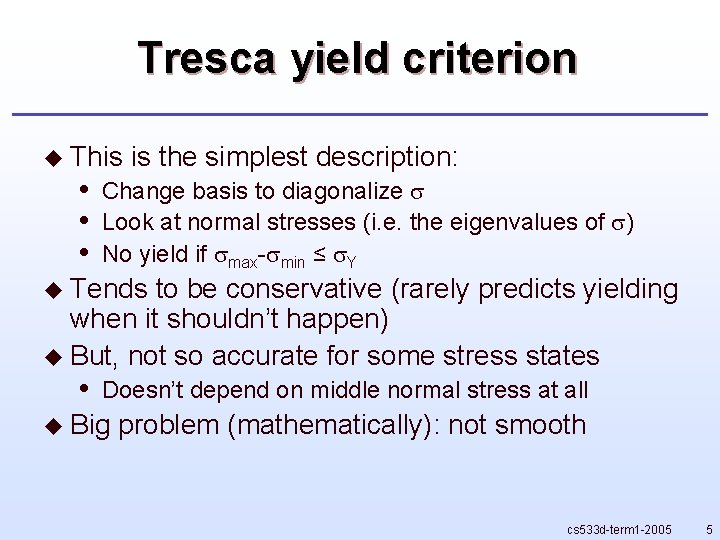Tresca yield criterion u This • • • is the simplest description: Change basis to diagonalize Look at normal stresses (i. e. the eigenvalues of ) No yield if max- min ≤ Y u Tends to be conservative (rarely predicts yielding when it shouldn’t happen) u But, not so accurate for some stress states • Doesn’t depend on middle normal stress at all u Big problem (mathematically): not smooth cs 533 d-term 1 -2005 5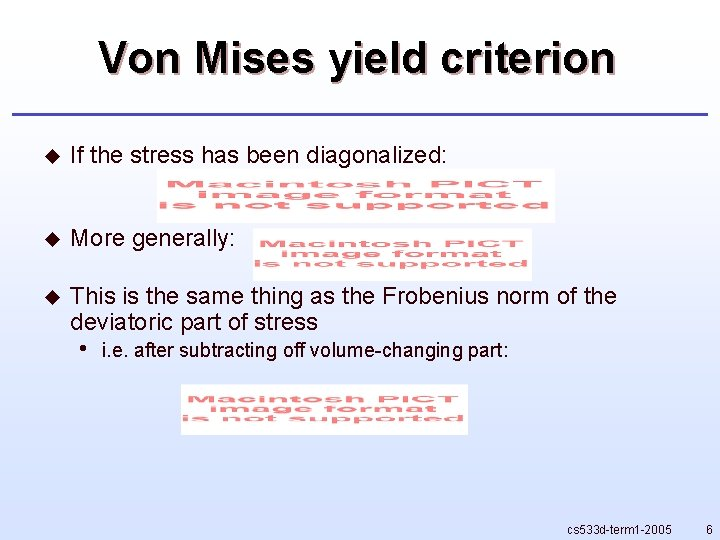Von Mises yield criterion u If the stress has been diagonalized: u More generally: u This is the same thing as the Frobenius norm of the deviatoric part of stress • i. e. after subtracting off volume-changing part: cs 533 d-term 1 -2005 6Linear elasticity shortcut u For linear (and isotropic) elasticity, apart from the volume-changing part which we cancel off, stress is just a scalar multiple of strain • (ignoring damping) u So can evaluate von Mises with elastic strain tensor too (and an appropriately scaled yield strain) cs 533 d-term 1 -2005 7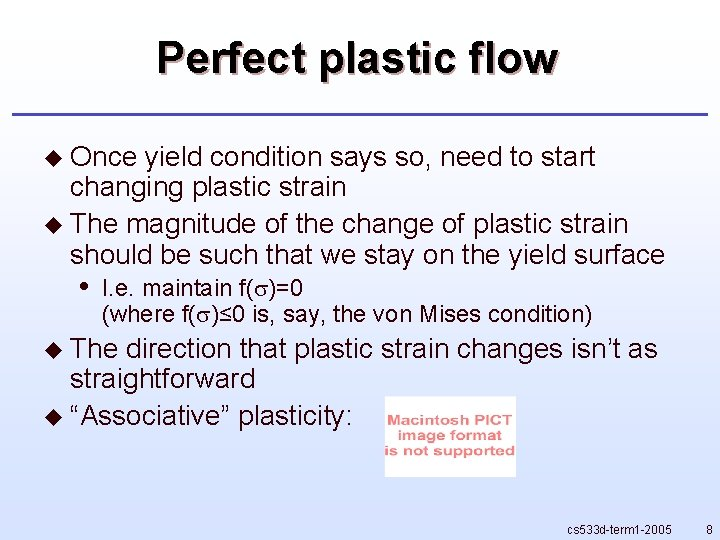Perfect plastic flow u Once yield condition says so, need to start changing plastic strain u The magnitude of the change of plastic strain should be such that we stay on the yield surface • I. e. maintain f( )=0 (where f( )≤ 0 is, say, the von Mises condition) u The direction that plastic strain changes isn’t as straightforward u “Associative” plasticity: cs 533 d-term 1 -2005 8Algorithm u After a time step, check von Mises criterion: is ? u If • so, need to update plastic strain: with chosen so that f( new)=0 (easy for linear elasticity) cs 533 d-term 1 -2005 9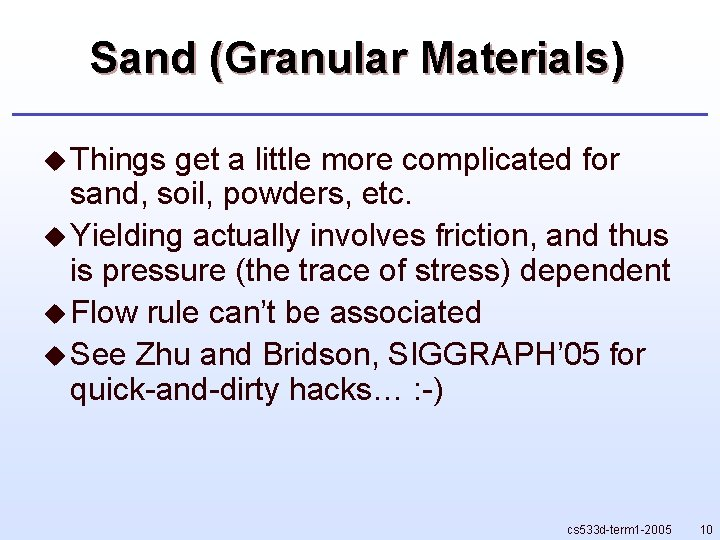Sand (Granular Materials) u Things get a little more complicated for sand, soil, powders, etc. u Yielding actually involves friction, and thus is pressure (the trace of stress) dependent u Flow rule can’t be associated u See Zhu and Bridson, SIGGRAPH’ 05 for quick-and-dirty hacks… : -) cs 533 d-term 1 -2005 10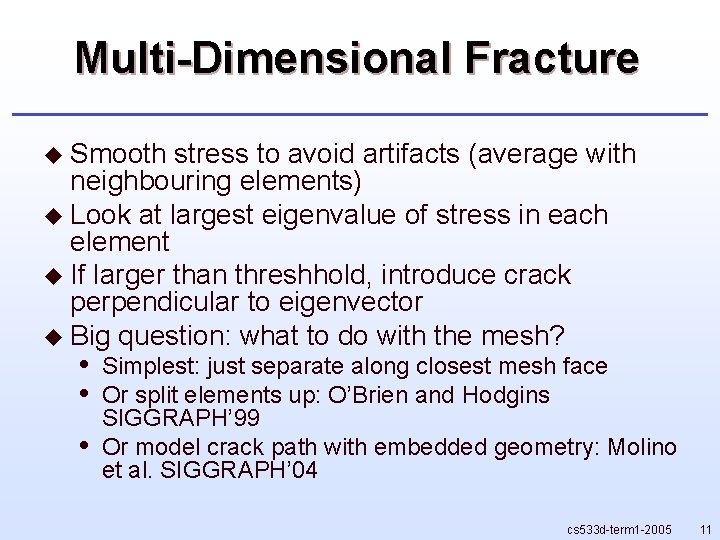Multi-Dimensional Fracture u Smooth stress to avoid artifacts (average with neighbouring elements) u Look at largest eigenvalue of stress in each element u If larger than threshhold, introduce crack perpendicular to eigenvector u Big question: what to do with the mesh? • • • Simplest: just separate along closest mesh face Or split elements up: O’Brien and Hodgins SIGGRAPH’ 99 Or model crack path with embedded geometry: Molino et al. SIGGRAPH’ 04 cs 533 d-term 1 -2005 11Fluids cs 533 d-term 1 -2005 12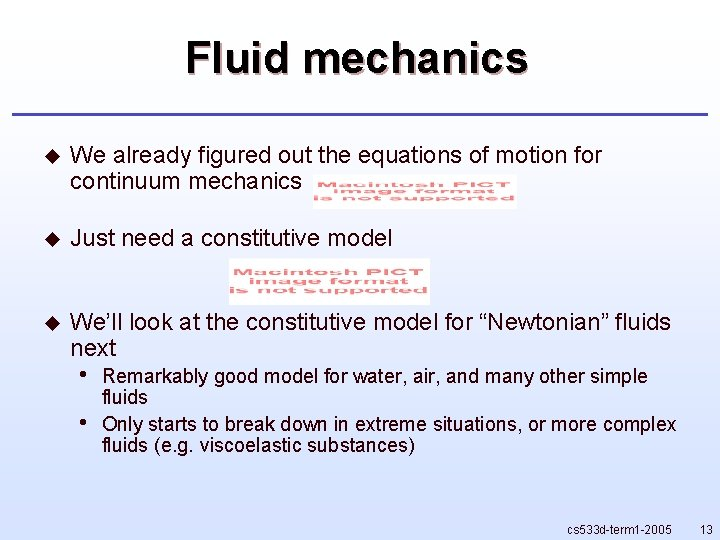Fluid mechanics u We already figured out the equations of motion for continuum mechanics u Just need a constitutive model u We’ll look at the constitutive model for “Newtonian” fluids next • • Remarkably good model for water, air, and many other simple fluids Only starts to break down in extreme situations, or more complex fluids (e. g. viscoelastic substances) cs 533 d-term 1 -2005 13Inviscid Euler model u u u Inviscid=no viscosity Great model for most situations • Numerical methods usually end up with viscosity-like error terms anyways… Constitutive law is very simple: • • • New scalar unknown: pressure p Barotropic flows: p is just a function of density (e. g. perfect gas law p=k( - 0)+p 0 perhaps) For more complex flows need heavy-duty thermodynamics: an equation of state for pressure, equation for evolution of internal energy (heat), … cs 533 d-term 1 -2005 14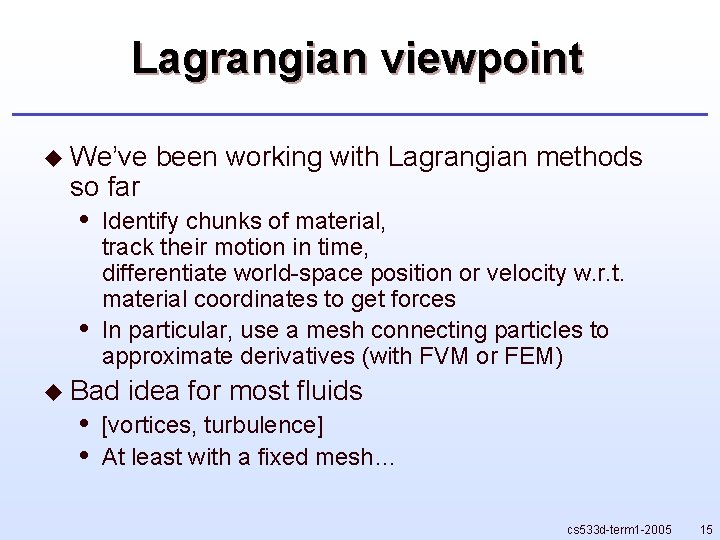Lagrangian viewpoint u We’ve so far • • Identify chunks of material, track their motion in time, differentiate world-space position or velocity w. r. t. material coordinates to get forces In particular, use a mesh connecting particles to approximate derivatives (with FVM or FEM) u Bad • • been working with Lagrangian methods idea for most fluids [vortices, turbulence] At least with a fixed mesh… cs 533 d-term 1 -2005 15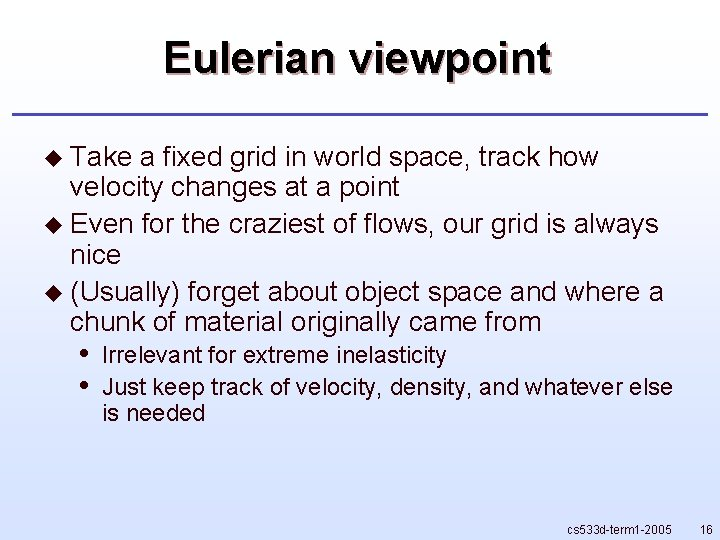Eulerian viewpoint u Take a fixed grid in world space, track how velocity changes at a point u Even for the craziest of flows, our grid is always nice u (Usually) forget about object space and where a chunk of material originally came from • • Irrelevant for extreme inelasticity Just keep track of velocity, density, and whatever else is needed cs 533 d-term 1 -2005 16Conservation laws u Identify any fixed volume of space u Integrate some conserved quantity in it (e. g. mass, momentum, energy, …) u Integral changes in time only according to how fast it is being transferred from/to surrounding space • Called the flux • [divergence form] cs 533 d-term 1 -2005 17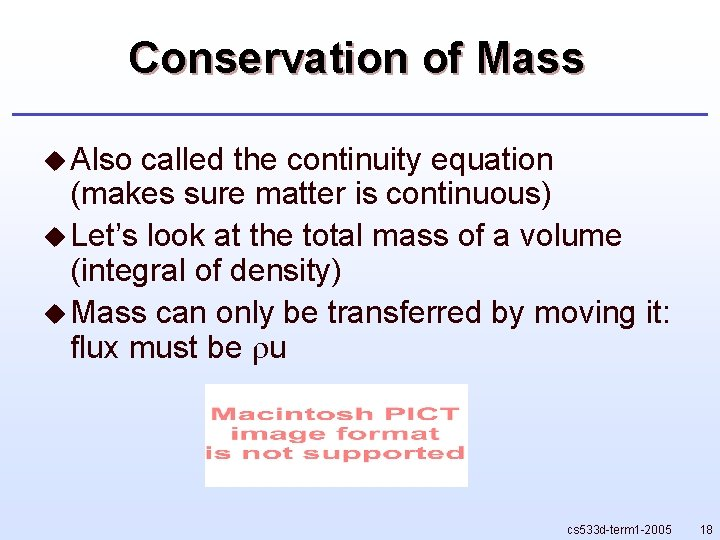Conservation of Mass u Also called the continuity equation (makes sure matter is continuous) u Let’s look at the total mass of a volume (integral of density) u Mass can only be transferred by moving it: flux must be u cs 533 d-term 1 -2005 18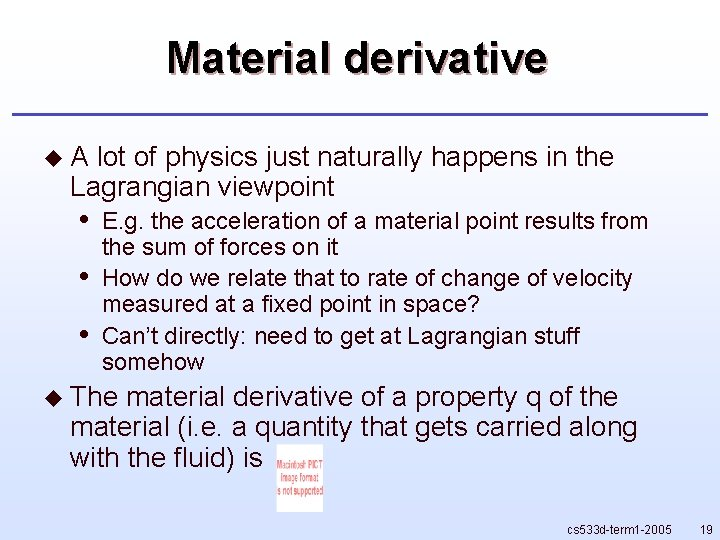Material derivative u. A lot of physics just naturally happens in the Lagrangian viewpoint • • • E. g. the acceleration of a material point results from the sum of forces on it How do we relate that to rate of change of velocity measured at a fixed point in space? Can’t directly: need to get at Lagrangian stuff somehow u The material derivative of a property q of the material (i. e. a quantity that gets carried along with the fluid) is cs 533 d-term 1 -2005 19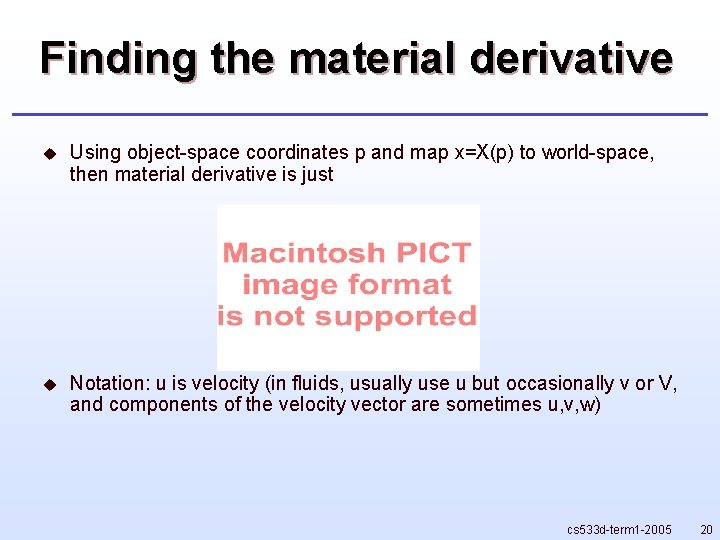Finding the material derivative u Using object-space coordinates p and map x=X(p) to world-space, then material derivative is just u Notation: u is velocity (in fluids, usually use u but occasionally v or V, and components of the velocity vector are sometimes u, v, w) cs 533 d-term 1 -2005 20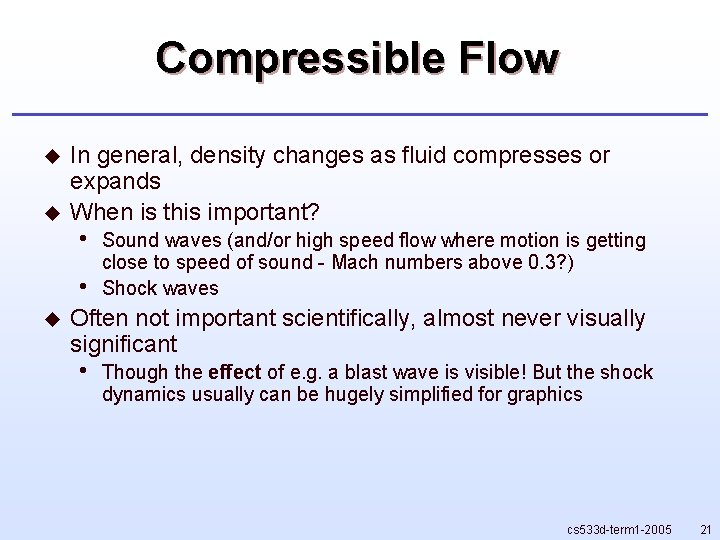Compressible Flow u u In general, density changes as fluid compresses or expands When is this important? • • u Sound waves (and/or high speed flow where motion is getting close to speed of sound - Mach numbers above 0. 3? ) Shock waves Often not important scientifically, almost never visually significant • Though the effect of e. g. a blast wave is visible! But the shock dynamics usually can be hugely simplified for graphics cs 533 d-term 1 -2005 21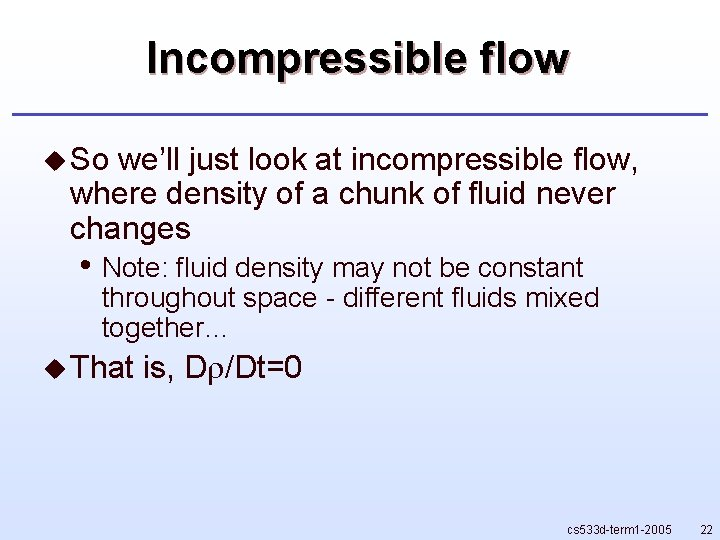Incompressible flow u So we’ll just look at incompressible flow, where density of a chunk of fluid never changes • Note: fluid density may not be constant throughout space - different fluids mixed together… u That is, D /Dt=0 cs 533 d-term 1 -2005 22Simplifying u Incompressibility: u Conservation u Subtract of mass: the two equations, divide by : u Incompressible • == divergence-free velocity Even if density isn’t uniform! cs 533 d-term 1 -2005 23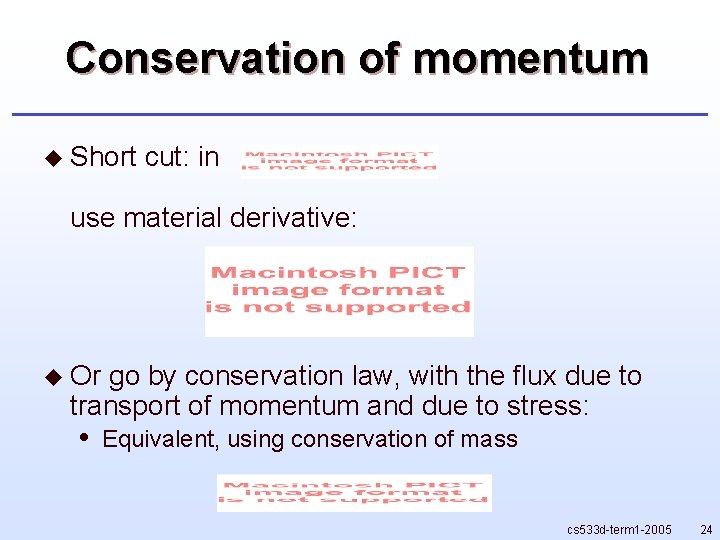Conservation of momentum u Short cut: in use material derivative: u Or go by conservation law, with the flux due to transport of momentum and due to stress: • Equivalent, using conservation of mass cs 533 d-term 1 -2005 24Inviscid momentum equation u Plug in simplest consitutive law ( =-p ) from before to get • Together with conservation of mass: the Euler equations cs 533 d-term 1 -2005 25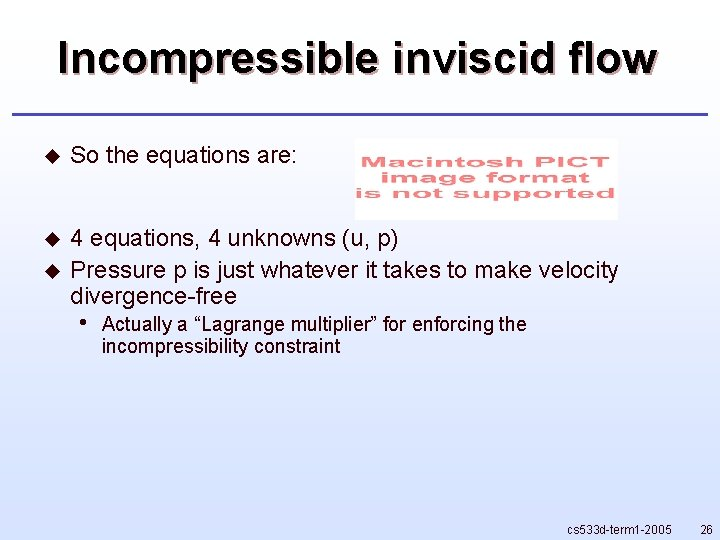Incompressible inviscid flow u So the equations are: u 4 equations, 4 unknowns (u, p) Pressure p is just whatever it takes to make velocity divergence-free u • Actually a “Lagrange multiplier” for enforcing the incompressibility constraint cs 533 d-term 1 -2005 26Pressure solve u To see what pressure is, take divergence of momentum equation u For constant density, just get Laplacian (and this is Poisson’s equation) u Important numerical methods use this approach to find pressure cs 533 d-term 1 -2005 27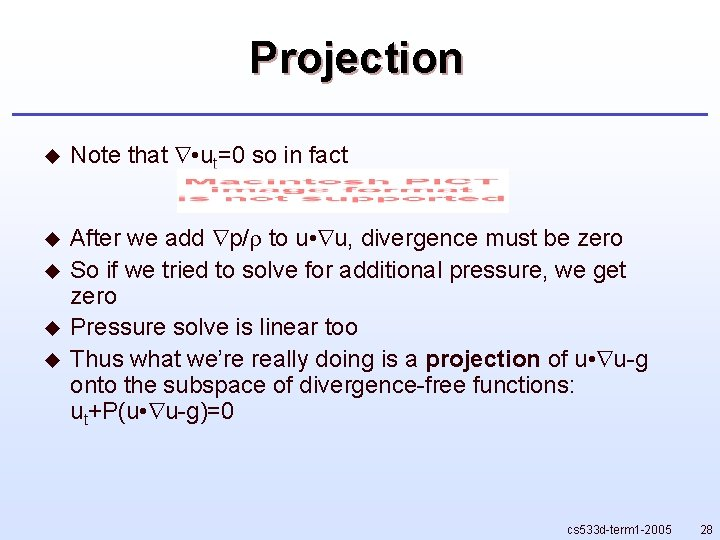Projection u Note that • ut=0 so in fact u After we add p/ to u • u, divergence must be zero So if we tried to solve for additional pressure, we get zero Pressure solve is linear too Thus what we’re really doing is a projection of u • u-g onto the subspace of divergence-free functions: ut+P(u • u-g)=0 u u u cs 533 d-term 1 -2005 28